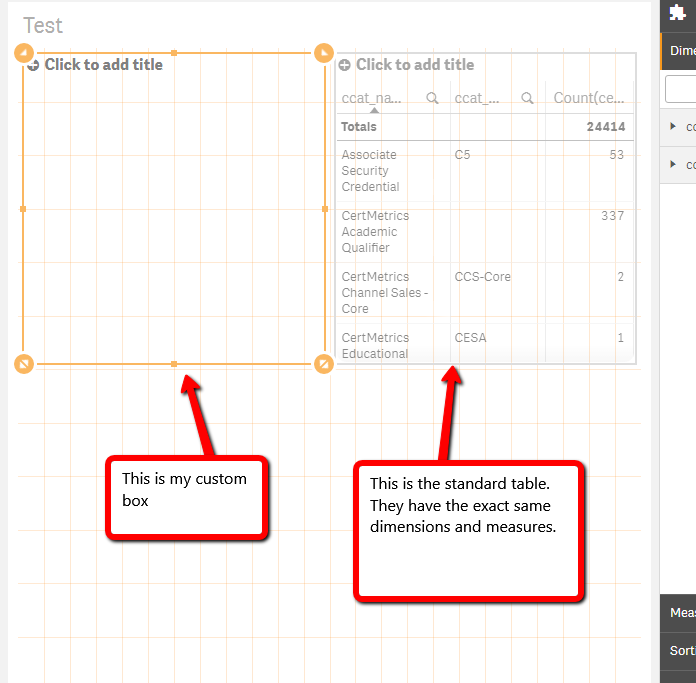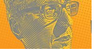# Integration, Extension & APIs

Announcements
QlikWorld starts MONDAY! last chance to register is now ! REGISTER NOW
cancel
Showing results for
Did you mean:Partner

## Tutorial on Hello Data not working

I'm following this example

http://qliksite.io/tutorials/qliksense-visualization-extensions/part-01/08-Hello-Data/#

I was writing it myself, but I literally copy and pasted your example to make sure I didn't have any typos. Whenever I try to access the hyper cube data, I get an error because I get not arrow objects in my qDataPages array, so therefor no qMatrix.

The error I get is thisHere is what the data looks like.So I'll post the code, but it's verbatim that project.

```define( [],
function ( ) {
'use strict';

return {

definition: {
type: "items",
component: "accordion",
items: {
dimensions: {
uses: "dimensions"
},
measures: {
uses: "measures"
},
sorting: {
uses: "sorting"
},
appearance: {
uses: "settings"
}
}
},
initialProperties: {

},
paint: function ( \$element, layout ) {

var hc = layout.qHyperCube;
console.log( 'Data returned: ', hc);

// Default rendering with HTML injection
\$element.empty();
var table = '<table border="1">';

table += '<tr>';
for (var i = 0; i < hc.qDimensionInfo.length; i++) {
table += '<th>' + hc.qDimensionInfo.qFallbackTitle + '</th>';
}
for (var i = 0; i < hc.qMeasureInfo.length; i++) {
table += '<th>' + hc.qMeasureInfo.qFallbackTitle + '</th>';
}
table += '</tr>';

table += '<tbody>';

for (var r = 0; r < hc.qDataPages.qMatrix.length; r++) {
table += '<tr>';
for (var c = 0; c < hc.qDataPages.qMatrix.length; c++) {
table += '<td>';
table += hc.qDataPages.qMatrix.qText;
table += '</td>';
}
table += '</tr>';
}
table += '</tbody>';
table += '</table>';
\$element.append( table );

}
};

} );
```
10 RepliesEmployee

‌Have a look at your initialProperties, they need to be defined (as also stated in the tutorial you are mentioning).

Hope this helps.

Regards

StefanPartner
Author

Sorry, it was just a bad copy and paste. I was playing around with stuff to see if I could get it to work.

initialProperties: {
qHyperCubeDef: {
qDimensions: [],
qMeasures: [],
qInitialDataFetch: [
{
qWidth: 10,
qHeight: 100
}
]
}
},

Doesn't solve anythingEmployee

Make sure you delete the object from the sheet and re-add it again if you have modified the initalProperties as they are set on object creation.Partner

Hi.

Im doing the exact tutorial as you are. In the tutorial it say that you should just add "initialProperties: {}," and that sense will use this to default itself to what is standard.

However when you do that, you get this exact issue. No data in the hypercube.

But when you manually add the code

initialProperties: {

qHyperCubeDef: {

qDimensions: [],

qMeasures: [],

qInitialDataFetch: [

{

qWidth: 10,

qHeight: 100

}

]

}

},

Then it works.

is this something that has been changed in later versions of sanse?Partner

Hi Michael,

I had exactly the same problem and found the solution while working further in the same tutorial.

Short explanation from what I have understood:

When using extra files like in this example './initialproperties', you need to create a parameter inside function(). Also, because initialdata is the second file I include, it also has to be the second parameter (at least that's what I think, I didn't test that). That parameter than has to be used instead of empty brackets.

Inside the files itself (here properties.js as well as initialproperties.js) you have to use

define( [], function () {

'use strict';

return {...}

}

and the inside of return{} is exactly what the belonging parameter will contain.

Hope this helps anybody ^^.

Michael

• Hello-Data.js:

define(

[

'./properties',

'./initialproperties'

],

function ( props, initProps ) {

'use strict';

return {

definition: props,

initialProperties: initProps,

paint: function ( \$element, layout ) {

var hc = layout.qHyperCube;

console.log( 'Data returned: ', hc );

\$element.empty();

var table = '<table border="1">';

table += '<tr>';

for (var i=0; i < hc.qDimensionInfo.length; i++){

table += '<th>' + hc.qDimensionInfo.qFallbackTitle + '</th>';

}

for (var i=0; i < hc.qMeasureInfo.length; i++){

table += '<th>' + hc.qMeasureInfo.qFallbackTitle + '</th>';

}

table += '</tr>';

table += '<tbody>';

// iterate over all rows

for (var r = 0; r < hc.qDataPages.qMatrix.length; r++) {

table += '<tr>';

// iterate over all cells within a row

for (var c = 0; c < hc.qDataPages.qMatrix.length; c++) {

table += '<td>';

table += hc.qDataPages.qMatrix.qText;

table += '</td>';

}

table += '</tr>';

}

table += '</tbody>';

table += '</table>';

\$element.append( table );

}

};

}

);

• properties.js:

define( [], function () {

'use strict';

var dimensions = {

uses: "dimensions"

};

var measures = {

uses: "measures"

};

var sorting = {

uses: "sorting"

};

var appearance = {

uses: "settings"

};

return {

type: "items",

component: "accordion",

items: {

dimensions: dimensions,

measures: measures,

sorting: sorting,

appearance: appearance

}

};

} );

• initialproperties.js:

define( [], function () {

'use strict';

return {

qHyperCubeDef: {

qDimensions: [],

qMeasures: [],

qInitialDataFetch: [

{

qWidth: 10,

qHeight: 50

}

]

}

};

} );Partner

It's still the case. Very frustrating that official tutorial is incorrect for a good couple of years alreadyEmployee

Please note that qliksite.io is a private site not a Qlik official site. It is where the widget concept was incubated before Qlik brought it into standard product.  Please reference the official Qlik help for supported documentation and tutorials on widgetsPartner

Ahh, sorry, haven't noticed the link in the first message and didn't know about qliksite.io. In my message I was referring to the official help page here https://help.qlik.com/en-US/sense-developer/April2019/Subsystems/Extensions/Content/Sense_Extensions...  in "Create the main script file" section Initial Properties is empty.Employee

Thank you for the clarification Yura. We will have this looked at. Apologies